## ISSB Mechanical Aptitude Test - 31. Which weight can slide down most easily?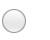ACBD2. Weight A, B, C and D are equal in size. Which winch can be most easily operated to lift the weight?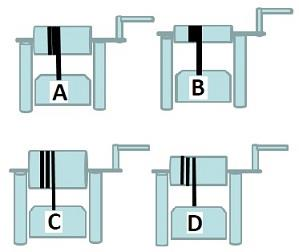CBDA3. What would be the current flowing from point "X", if another power source is attached as shown?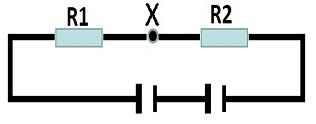Current flow will doubleEither no change or halvedCurrent flow will not changeCurrent flow will be halved4. Which vehicle/s cannot reverse due to direction of their rear wheels?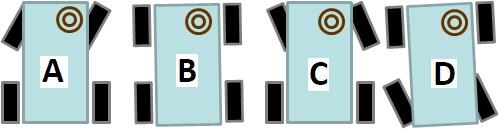AD and BBC5. Which bottle is heaviest?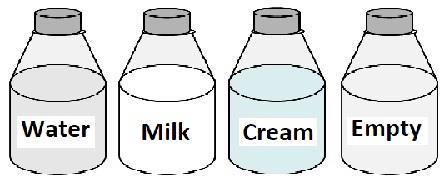CreamWaterMilkEmpty6. On which black pole the weight pushes harder?CBEqual on all polesA7. If Cog D turns anti clockwise, which other cogs turn anticlockwise?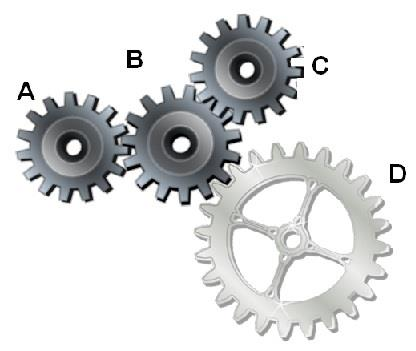A, B and CA and CA and BB and C8. At which point the pendulem speed will be maximum?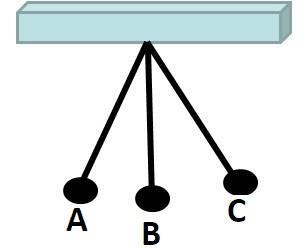CA and CAB9. Weight A, B, C and D are equal in size. Which winch is most difficult to operate to lift the weight?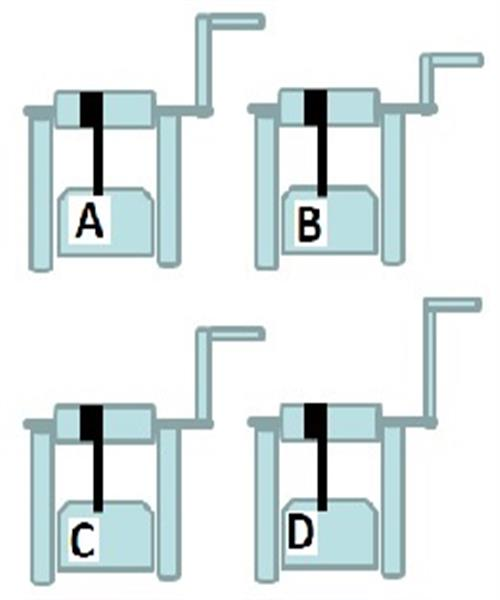CADB10. If Switch "A and B" is switched on, which bulbs will be lit?4, 5 and 63 and 63, 5 and 63 and 411. What would be the current flowing from point "X", if R2 is removed?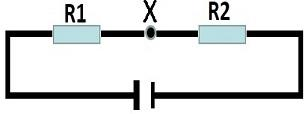Current flow will not changeEither no change or halvedCurrent flow will be halvedCurrent flow will double

This is more feedback!
This is the feedback!